### Home > MC2 > Chapter 6 > Lesson 6.1.4 > Problem6-40

6-40.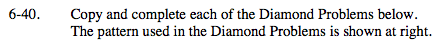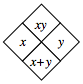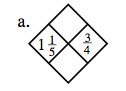Converting the mixed number into a fraction greater than one may prove to be helpful.

$\left(\frac{6}{5}\right)\left(\frac{3}{4}\right) = ?$

$\frac{6}{5} + \frac{3}{4} = ?$

${ \text{Top: }\frac{9}{10}\\\;\;\text{Bottom: }\frac{39}{20}}$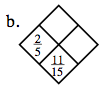$\frac{2}{5} + ? = \frac{11}{15}$

${ \text{Top: }\frac{2}{15}\\\;\;\text{Right: }\frac{1}{3}}$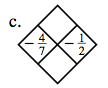Use the method presented in part (a).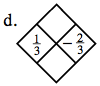Use the method presented in part (a).